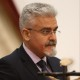Hello friends, I hope you all are doing great. In today’s tutorial, I am going to show you How to Interface Arduino with 74HC595 & 74HC165. I have already interfaced these shift registers separately with Arduino. In the first tutorial we have seen Arduino 74HC595 Interfacing in which I have discussed How to increase the output pins of Arduino using 74HC595. After that in second tutorial we have seen Arduino 74HC165 Interfacing where we have increased the input pins of Arduino.

So, now we are gonna interface both of these shift registers with Arduino UNO and will increase both input and output pins of Arduino. I have also given the Proteus simulations for download at the end of this tutorial along with Arduino code. So, lets get started with Interfacing of Arduino with 74HC595 & 74HC165:

#### Interfacing of Arduino with 74HC595 & 74HC165• As you can see in above figure, I have used 74HC165 & 74HC595 and interfaced its pins with Arduino UNO.
• I could use same clock for these shift registers but it would have made the code quite complex.
• That’s why I have used separate clock pins and I have used the below code to reflect the input on output.
```#define NUMBER_OF_SHIFT_CHIPS   1
#define DATA_WIDTH   NUMBER_OF_SHIFT_CHIPS * 8
#define TotalIC 2
#define TotalICPins TotalIC * 8

int EnablePin  = 9;
int DataPin    = 11;
int ClockPin   = 12;

int RCLK = 5;
int SER = 6;
int SRCLK = 7;

unsigned long pinValues;
unsigned long oldPinValues;
boolean Data[TotalICPins];

void setup()
{
Serial.begin(9600);

pinMode(EnablePin, OUTPUT);
pinMode(ClockPin, OUTPUT);
pinMode(DataPin, INPUT);

digitalWrite(ClockPin, LOW);
Serial.println("Visit us at www.TheEngineeringProjects.com");
Serial.println();
pinMode(SER, OUTPUT);
pinMode(RCLK, OUTPUT);
pinMode(SRCLK, OUTPUT);

ClearBuffer();

print_byte();
oldPinValues = pinValues;
}

void loop()
{

if(pinValues != oldPinValues)
{
print_byte();
oldPinValues = pinValues;
}

}

{
long bitVal;
unsigned long bytesVal = 0;

digitalWrite(EnablePin, HIGH);
delayMicroseconds(5);
digitalWrite(EnablePin, LOW);

for(int i = 0; i < DATA_WIDTH; i++)
{
bytesVal |= (bitVal << ((DATA_WIDTH-1) - i));

digitalWrite(ClockPin, HIGH);
delayMicroseconds(5);
digitalWrite(ClockPin, LOW);
}

return(bytesVal);
}

void print_byte() {
byte i;

Serial.println("*Shift Register Values:*\r\n");

for(byte i=0; i<=DATA_WIDTH-1; i++)
{
Serial.print("P");
Serial.print(i+1);
Serial.print(" ");
}
Serial.println();
for(byte i=0; i<=DATA_WIDTH-1; i++)
{

Serial.print(pinValues >> i & 1, BIN);
Data[i] = pinValues >> i & 1, BIN;
//if(BinaryValue == 1){Data[i] = HIGH;}
//if(BinaryValue == 0){Data[i] = LOW;}
UpdateData();
if(i>8){Serial.print(" ");}
Serial.print("  ");

}

Serial.print("\n");
Serial.println();Serial.println();

}

void ClearBuffer()
{
for(int i = TotalICPins - 1; i >=  0; i--)
{
Data[i] = LOW;
}
UpdateData();
}

void UpdateData()
{
digitalWrite(RCLK, LOW);
for(int i = TotalICPins - 1; i >=  0; i--)
{
digitalWrite(SRCLK, LOW);
digitalWrite(SER, Data[i]);
digitalWrite(SRCLK, HIGH);

}
digitalWrite(RCLK, HIGH);
}```
• In the above code, I have used Number_of_Shift_Chips 1 and it means I am using 1 chip each, so in total 2 chips.
• Now get hex file from Arduino software and upload it in your Proteus software.
• Run your simulation and if everything goes fine then you will get something as shown in below figure:• You can see in above figure that all those LED outputs are ON which has HIGH inputs.
• I have also attached a Virtual Terminal with Arduino to have a look at the input bits.
• Now let’s add 2 chips of 74HC165 and 74HC959, so design a simple simulation as shown in below figure:• Now in your above code change the Number of Shift chips from 1 to 2, as now we are using 2 chips each.
• Upload your hex file and if everything goes fine then you will get similar results:• So, that’s how you can easily increase input and output pins of Arduino UNO.
• I have just designed a simple code but you can work on it and can control these inputs separately as well.
• You can interface different digital sensors on these input pins and can control motors, relays, solenoids etc. at output pins.
• You can download both of these Proteus Simulations along with Arduino code by clicking the below button, but I would suggest you to dwsign it on yoru own so that you could learn from mistakes.

So, that was all about Interfacing of Arduino with 74HC595 & 74HC165. I hope you can now easily simulate it. If you have any questions then ask in comments and I will try my best to resolve them. Thanks for reading. Take care !!! 🙂

1.Juan-Ramón Muñoz Rico says:

Hi friend!

My code only works with 4 chips, despite changing

#define NUMBER_OF_SHIFT_CHIPS 1
#define TotalIC 2

to

#define NUMBER_OF_SHIFT_CHIPS 6
#define TotalIC 6

because I have 6 165 chips and 6 595 chips.

What do I do wrong?

• Don van Raak says:

Hello,
I think it must be,

#define NUMBER_OF_SHIFT_CHIPS 5
#define TotalIC 10

if you are using 5 of each IC.

But still only 4 of them work. So I am also curious for the answer. I have made seperate 2 boards, one for the inputs and one for the outputs.
If I upload an other sketch to try the 74hc595 outputs the 5 of them work. So I think I have put it together correctly.